Courses

# Introduction to Power Plant & Steam Cycle - MCQ Test 2

## 30 Questions MCQ Test RRB JE for Mechanical Engineering | Introduction to Power Plant & Steam Cycle - MCQ Test 2

Description
This mock test of Introduction to Power Plant & Steam Cycle - MCQ Test 2 for Mechanical Engineering helps you for every Mechanical Engineering entrance exam. This contains 30 Multiple Choice Questions for Mechanical Engineering Introduction to Power Plant & Steam Cycle - MCQ Test 2 (mcq) to study with solutions a complete question bank. The solved questions answers in this Introduction to Power Plant & Steam Cycle - MCQ Test 2 quiz give you a good mix of easy questions and tough questions. Mechanical Engineering students definitely take this Introduction to Power Plant & Steam Cycle - MCQ Test 2 exercise for a better result in the exam. You can find other Introduction to Power Plant & Steam Cycle - MCQ Test 2 extra questions, long questions & short questions for Mechanical Engineering on EduRev as well by searching above.
QUESTION: 1

Solution:
QUESTION: 2

Solution:
QUESTION: 3

### Consider a steam power plant using a reheat cycle as shown. Steam leaves the boiler and enters the turbine at 4 MPa, 350°C (h3 = 3095 kJ/kg). After expansion in the turbine to 400 kPa (h4 = 2609 kJ/kg), the steam is reheated to 350°C (h5 = 3170 kJ / kg), and then expanded in a low pressure turbine to 10 kPa (h6= 2165 kJ/kg). The specific volume of liquid handled by the pump can be assumed to be .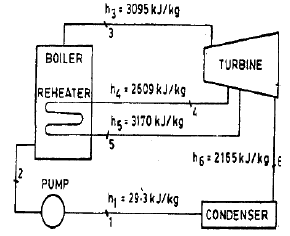The thermal efficiency of the plant neglecting pump work is

Solution: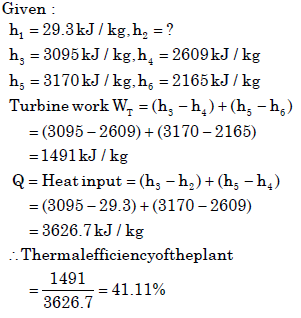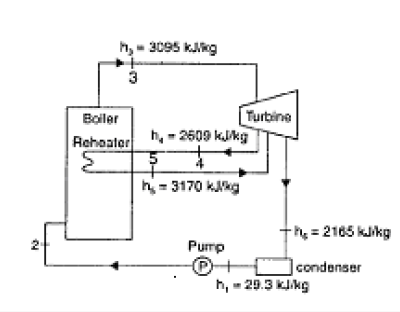QUESTION: 4

Consider a steam power plant using a reheat cycle as shown. Steam leaves the boiler and enters the turbine at 4 MPa, 350°C (h3 = 3095 kJ/kg). After expansion in the turbine to 400 kPa (h4 = 2609 kJ/kg), the steam is reheated to 350°C (h5 = 3170 kJ / kg), and then expanded in a low pressure turbine to 10 kPa (h6= 2165 kJ/kg). The specific volume of liquid handled by the pump can be assumed to be .The enthalpy at the pump discharge (h2) is

Solution:

Enthalpy at the pump discharge will be greater than 29.3 kJ/kg Hence from given choice clearly we can say h2 = 33.3kJ / kg

QUESTION: 5

12. A steam plant has the boiler efficiency of 92%, turbine efficiency (mechanical) of 94%, generator efficiency of 95% and cycle efficiency of44%. If 6% of the generated power is used to run the auxiliaries, the overallplant efficiency is

Solution: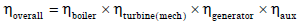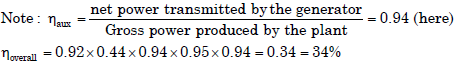QUESTION: 6

In steam and other vapour cycles, the process of removing noncondensableis called

Solution:
QUESTION: 7

Which one of the following statements is correct?

Solution:

Carnot cycle has highestefficiency but very high back. So work ratio is low.

QUESTION: 8

During which of following process does heat rejection take place in Carnotvapour cycle?

Solution:
QUESTION: 9

The main advantage of a reheat Rankine cycle is

Solution:
QUESTION: 10

Assertion (A): The performance of a simple Rankine cycle is not sensitive to the
efficiency of the feed pump.

Reason (R): The net work ratio is practically unity for a Rankine cycle

Solution:
QUESTION: 11

The reheat cycle in steam power plant is mainly adopted to

Solution:
QUESTION: 12

Assertion (A): The purpose of employing reheat in a steam power plant is mainlyto improve its thermal efficiency.Reason (R): The use of regeneration in a steam power plant improves theefficiency.

Solution:
QUESTION: 13

Consider the following statements:If steam is reheated during the expansion through turbine stages
1. Erosion of blade will decrease
2. The overall pressure ratio will increase.
3. The total heat drop will increase.Of these statements

Solution:

Overall pressure ratio depends on inlet pressure and condenser pressure

QUESTION: 14

Assertion (A): An ideal regenerative Ranking cycle power plant with saturated steam at   the inlet to the turbine  has  same  thermal  efficiency as Carnot cycleworking between the same temperature limits .  Reason (R): The change in entropy of steam during expansion in the turbine is equal to the change in entropy of the feed water during sensible heating at steam generator pressure.

Solution:
QUESTION: 15

(A): The thermal efficiency of a regenerative Rankine cycle is always higher than that of a cycle without regeneration.Reason (R): In regeneration cycle the work output is more

Solution:

Thermal efficiency of Regenerating Rankine cycle is higher than without regeneration. However, work output is less due to partial extraction of steam. Thus A is true but R is false

QUESTION: 16

In a steam power plant, the ratio of the isentropic heat drop in the primemover to the amount of heat supplied per unit mass of steam is known as

Solution:
QUESTION: 17

Assertion (A): Rankine efficiency would approach Carnot cycle efficiency byproviding a series of regenerative feed heating.Reason (R): With regenerative feed heating, expansion through the turbine approaches an isentropic process.

Solution:
QUESTION: 18

A regenerative steam cycle renders

Solution:

In regenerative steam cycle. a part of steam is extracted from turbine and utilized to heat up condensate. In this way some work is lost per unit mass of steam corresponding to steam extracted out, but its heat is not wasted to cooling water but
conserved within the cycle thus increasing thermal efficiency.

QUESTION: 19

When is the greatest economy obtained in a regenerative feed heatingcycle?

Solution:
QUESTION: 20

In a regenerative feed heating cycle, the economic number of the stages of regeneration

Solution:

Since efficiency is proportional to gain in feed water temperature. As initial temperature and pressure increases the gain in feed water temperature decreases i.e. efficiency gain follows the law of diminishing return with increase in the
number of heaters.

QUESTION: 21

Which one of the following statements is not correct for a regenerative steam cycle?

Solution:
QUESTION: 22

In which one of the following steam turbines, steam is taken from various points along the turbine, solely for feed water heating?

Solution:

Note: Regenerative cycle not regenerative turbine so choice is ‘b’

QUESTION: 23

The temperature-entropy diagram for a steam turbine power plant, operating on the Rankine cycle with reheat and regenerative feed heating is shown in the given figure. If m denotes the fraction of steam bled for feed heating, the work developed in the turbine per kg steam entering the turbine at state 5 is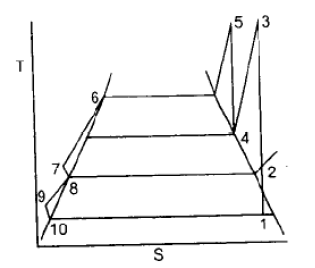Solution:
QUESTION: 24

In thermal power plants, the deaerator is used mainly to

Solution:

In thermal power plants, the deaerator is used mainly to remove dissolved gases from feed water

QUESTION: 25

Match List I with List II and select the correct answer: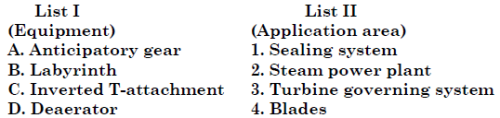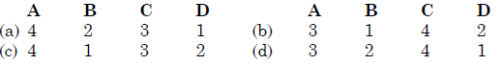Solution:
QUESTION: 26

Consider the following statements:The efficiency of the vapour power Rankine cycle can be increased by

1. Increasing the temperature of the working fluid at which heat is added.

2. Increasing the pressure of the working fluid at which heat is added.

3. Decreasing the temperature of the working fluid at which heat isrejected.

Which of these statements is/are correct?

Solution:
QUESTION: 27

Assertion (A): Rankine cycle is preferred for waste heat recovery.Reason (R): Rankine cycle gives high thermal efficiency even at low temperature scompared to other dynamic energy conversion systems

Solution:
QUESTION: 28

The efficiency of Rankine cycle is lower than that corresponding Carnotcycle because [IES-1992]

Solution:
QUESTION: 29

In the bottoming cycle of cogeneration, low grade waste heat is used for

Solution:
QUESTION: 30

A power plant, which uses a gas turbine followed by steam turbine for power generation, is called:

Solution: